Chemistry

# Chapter 15

ChemistryChapter 15

1.

(a) $AgI(s)⇌Ag+(aq)+I−(aq)xx_AgI(s)⇌Ag+(aq)+I−(aq)xx_$
(b) $CaCO3(s)⇌Ca2+(aq)+CO32−(aq)x_xCaCO3(s)⇌Ca2+(aq)+CO32−(aq)x_x$
(c) $Mg(OH)2(s)⇌Mg2+(aq)+2OH−(aq)x2x_Mg(OH)2(s)⇌Mg2+(aq)+2OH−(aq)x2x_$
(d) $Mg3(PO4)2(s)⇌3Mg2+(aq)+2PO43−(aq)3x_2xMg3(PO4)2(s)⇌3Mg2+(aq)+2PO43−(aq)3x_2x$
(e) $Ca5(PO4)3OH(s)⇌5Ca2+(aq)+3PO43−(aq)+OH−(aq)5x_3x_xCa5(PO4)3OH(s)⇌5Ca2+(aq)+3PO43−(aq)+OH−(aq)5x_3x_x$

3.

There is no change. A solid has an activity of 1 whether there is a little or a lot.

5.

The solubility of silver bromide at the new temperature must be known. Normally the solubility increases and some of the solid silver bromide will dissolve.

7.

CaF2, MnCO3, and ZnS

9.

(a) $LaF3(s)⇌La3+(aq)+3F−(aq)Ksp=[La3+][F−]3;LaF3(s)⇌La3+(aq)+3F−(aq)Ksp=[La3+][F−]3;$
(b) $CaCO3(s)⇌Ca2+(aq)+CO32−(aq)Ksp=[Ca2+][CO32−];CaCO3(s)⇌Ca2+(aq)+CO32−(aq)Ksp=[Ca2+][CO32−];$
(c) $Ag2SO4(s)⇌2Ag+(aq)+SO42−(aq)Ksp=[Ag+]2[SO42−];Ag2SO4(s)⇌2Ag+(aq)+SO42−(aq)Ksp=[Ag+]2[SO42−];$
(d) $Pb(OH)2(s)⇌Pb2+(aq)+2OH−(aq)Ksp=[Pb2+][OH−]2Pb(OH)2(s)⇌Pb2+(aq)+2OH−(aq)Ksp=[Pb2+][OH−]2$

11.

(a)1.77 $××$ 10–7; (b) 1.6 $××$ 10–6; (c) 2.2 $××$ 10–9; (d) 7.91 $××$ 10–22

13.

(a) 2 $××$ 10–2 M; (b) 1.5 $××$ 10–3 M; (c) 2.27 $××$ 10–9 M; (d) 2.2 $××$ 10–10 M

15.

(a) 6.4 $××$ 10−9 M = [Ag+], [Cl] = 0.025 M
Check: $6.4×10−9M0.025M×100%=2.6×10−5%,6.4×10−9M0.025M×100%=2.6×10−5%,$an insignificant change;
(b) 2.2 $××$ 10−5 M = [Ca2+], [F] = 0.0013 M
Check: $2.26×10−5M0.00133M×100%=1.70%.2.26×10−5M0.00133M×100%=1.70%.$ This value is less than 5% and can be ignored.
(c) 0.2238 M = $[SO42−];[SO42−];$ [Ag+] = 7.4 $××$ 10–3 M
Check: $3.7×10−30.2238×100%=1.64×10−2;3.7×10−30.2238×100%=1.64×10−2;$ the condition is satisfied.
(d) [OH] = 2.8 $××$ 10–3 M; 5.7 $××$ 10−12 M = [Zn2+]
Check: $5.7×10−122.8×10−3×100%=2.0×10−7%;5.7×10−122.8×10−3×100%=2.0×10−7%;$ x is less than 5% of [OH] and is, therefore, negligible.

17.

(a) [Cl] = 7.6 $××$ 10−3 M
Check: $7.6×10−30.025×100%=30%7.6×10−30.025×100%=30%$
This value is too large to drop x. Therefore solve by using the quadratic equation:
[Ti+] = 3.1 $××$ 10–2 M
[Cl] = 6.1 $××$ 10–3
(b) [Ba2+] = 7.7 $××$ 10–4 M
Check: $7.7×10−40.0313×100%=2.4%7.7×10−40.0313×100%=2.4%$
Therefore, the condition is satisfied.
[Ba2+] = 7.7 $××$ 10–4 M
[F] = 0.0321 M;
(c) Mg(NO3)2 = 0.02444 M
$[C2O42−]=2.9×10−5[C2O42−]=2.9×10−5$
Check: $2.9×10−50.02444×100%=0.12%2.9×10−50.02444×100%=0.12%$
The condition is satisfied; the above value is less than 5%.
$[C2O42−]=2.9×10−5M[C2O42−]=2.9×10−5M$
[Mg2+] = 0.0244 M
(d) [OH] = 0.0501 M
[Ca2+] = 3.15 $××$ 10–3
Check: $3.15×10−30.050×100%=6.28%3.15×10−30.050×100%=6.28%$
This value is greater than 5%, so a more exact method, such as successive approximations, must be used.
[Ca2+] = 2.8 $××$ 10–3 M
[OH] = 0.053 $××$ 10–2 M

19.

The changes in concentration are greater than 5% and thus exceed the maximum value for disregarding the change.

21.

CaSO4∙2H2O is the most soluble Ca salt in mol/L, and it is also the most soluble Ca salt in g/L.

23.

4.8 $××$ 10–3 M = $[SO42−][SO42−]$ = [Ca2+]; Since this concentration is higher than 2.60 $××$ 10–3 M, “gyp” water does not meet the standards.

25.

Mass (CaSO4·2H2O) = 0.72 g/L

27.

(a) [Ag+] = [I] = 1.3 $××$ 10–5 M; (b) [Ag+] = 2.88 $××$ 10–2 M, $[SO42−][SO42−]$= 1.44 $××$ 10–2 M; (c) [Mn2+] = 3.7 $××$ 10–5 M, [OH] = 7.4 $××$ 10–5 M; (d) [Sr2+] = 4.3 $××$ 10–2 M, [OH] = 8.6 $××$ 10–2 M; (e) [Mg2+] = 1.3 $××$ 10–4 M, [OH] = 2.6 $××$ 10–4 M.

29.

(a) 1.7 $××$ 10–4; (b) 8.2 $××$ 10–55; (c) 1.35 $××$ 10–4; (d) 1.18 $××$ 10–5; (e) 1.08 $××$ 10–10

31.

(a) CaCO3 does precipitate.
(b) The compound does not precipitate.
(c) The compound does not precipitate.
(d) The compound precipitates.

33.

3.03 $××$ 10−7 M

35.

9.2 $××$ 10−13 M

37.

[Ag+] = 1.8 $××$ 10–3 M

39.

6.3 $××$ 10–4

41.

(a) 2.25 L; (b) 7.2 $××$ 10–7 g

43.

100% of it is dissolved

45.

(a) $Hg22+Hg22+$ and Cu2+: Add $SO42−.SO42−.$
(b) $SO42−SO42−$ and Cl: Add Ba2+.
(c) Hg2+ and Co2+: Add S2–.
(d) Zn2+ and Sr2+: Add OH until [OH] = 0.050 M.
(e) Ba2+ and Mg2+: Add $SO42−.SO42−.$
(f) $CO32−CO32−$ and OH: Add Ba2+.

47.

AgI will precipitate first.

49.

1.5 $××$ 10−12 M

51.

3.99 kg

53.

(a) 3.1 $××$ 10–11; (b) [Cu2+] = 2.6 $××$ 10–3; $[IO3−][IO3−]$ = 5.3 $××$ 10–3

55.

1.8 $××$ 10–5 g Pb(OH)2

57.

$Mg(OH)2(s)⇌Mg2++2OH−Ksp=[Mg2+][OH−]2Mg(OH)2(s)⇌Mg2++2OH−Ksp=[Mg2+][OH−]2$
1.23 $××$ 10−3 g Mg(OH)2

59.

MnCO3 will form first, since it has the smallest Ksp value it is the least soluble. MnCO3 will be the last to precipitate, it has the largest Ksp value.

61.

when the amount of solid is so small that a saturated solution is not produced

63.

8 $××$ 10–5 M

65.

5 $××$ 1023

67.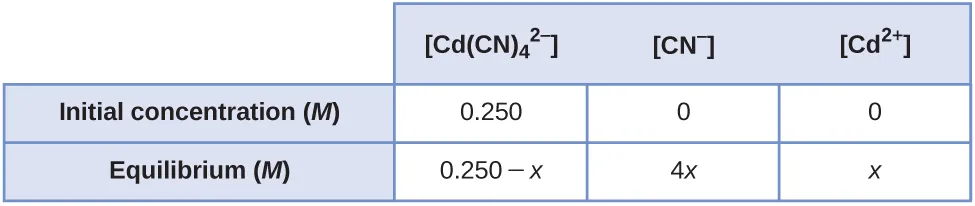[Cd2+] = 9.5 $××$ 10–5 M; [CN] = 3.8 $××$ 10–4 M

69.

[Co3+] = 3.0 $××$ 10–6 M; [NH3] = 1.8 $××$ 10–5 M

71.

1.3 g

73.

0.79 g

75.

(a)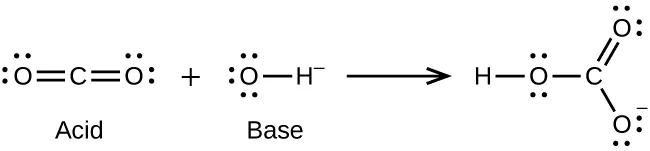;
(b)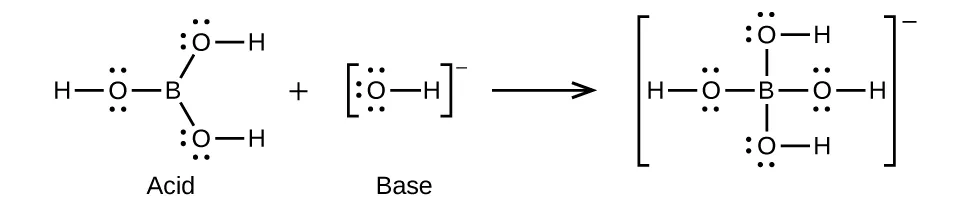;
(c)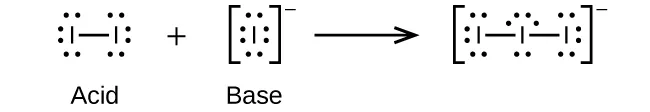;
(d)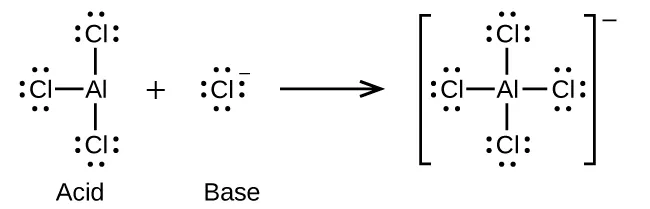;
(e)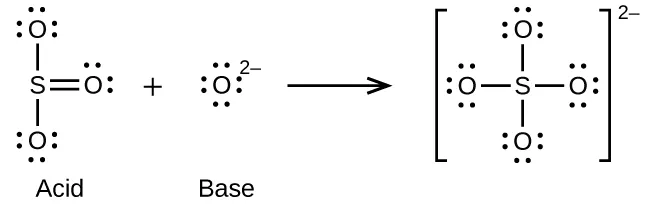77.

(a)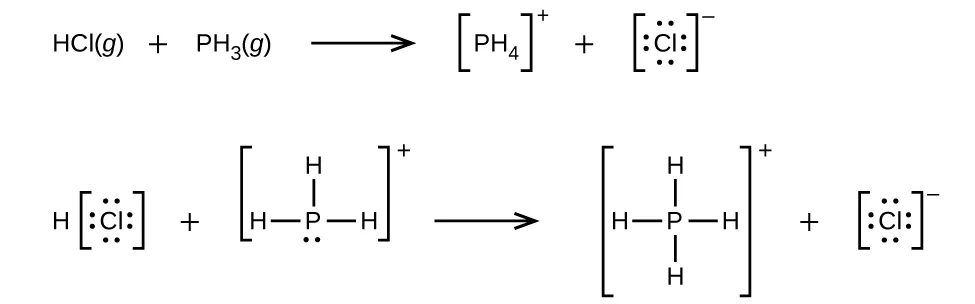;
(b) $H3O++CH3−⟶CH4+H2OH3O++CH3−⟶CH4+H2O$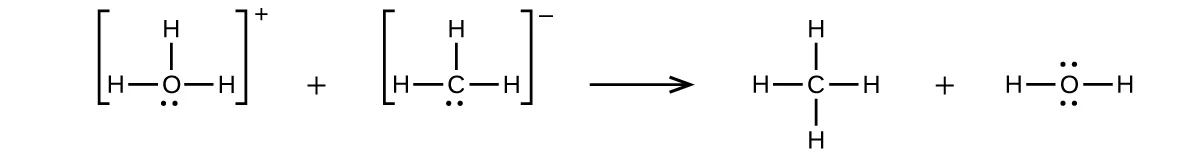;
(c) $CaO+SO3⟶CaSO4CaO+SO3⟶CaSO4$;
(d) $NH4++C2H5O−⟶C2H5OH+NH3NH4++C2H5O−⟶C2H5OH+NH3$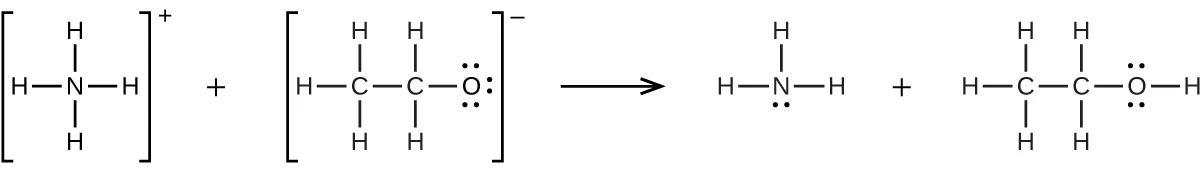79.

0.0281 g

81.

$HNO3(l)+HF(l)⟶H2NO3++F−;HNO3(l)+HF(l)⟶H2NO3++F−;$ $HF(l)+BF3(g)⟶H++BF4HF(l)+BF3(g)⟶H++BF4$

83.

(a) $H3BO3+H2O⟶H4BO4−+H+;H3BO3+H2O⟶H4BO4−+H+;$ (b) The electronic and molecular shapes are the same—both tetrahedral. (c) The tetrahedral structure is consistent with sp3 hybridization.

85.

0.014 M

87.

7.2 $××$ 10–15 M

89.

4.4 $××$ 10−22 M

91.

6.2 $××$ 10–6 M = [Hg2+]; 1.2 $××$ 10–5 M = [Cl]; The substance is a weak electrolyte because very little of the initial 0.015 M HgCl2 dissolved.

93.

[OH] = 4.5 $××$ 10−5; [Al3+] = 2.2 $××$ 10–20 (molar solubility)

95.

$[SO42−]=0.049M[SO42−]=0.049M$
[Ba2+] = 4.7 $××$ 10–7 (molar solubility)

97.

[OH] = 7.6 $××$ 10−3 M
[Pb2+] = 2.1 $××$ 10–11 (molar solubility)

99.

7.66

101.

$[CO32−]=0.116M[CO32−]=0.116M$
[Cd2+] = 4.5 $××$ 10−11 M

103.

3.1 $××$ 10−3 M

105.

0.0102 L (10.2 mL)

107.

0.0036 g

109.

(a) Ksp = [Mg2+][F]2 = (1.21 $××$ 10–3)(2 $××$ 1.21 $××$ 10–3)2 = 7.09 $××$ 10–9; (b) 7.09 $××$ 10–7 M
(c) Determine the concentration of Mg2+ and F that will be present in the final volume. Compare the value of the ion product [Mg2+][F]2 with Ksp. If this value is larger than Ksp, precipitation will occur.
0.1000 L $××$ 3.00 $××$ 10–3 M Mg(NO3)2 = 0.3000 L $××$ M Mg(NO3)2
M Mg(NO3)2 = 1.00 $××$ 10–3 M
0.2000 L $××$ 2.00 $××$ 10–3 M NaF = 0.3000 L $××$ M NaF
M NaF = 1.33 $××$ 10–3 M
ion product = (1.00 $××$ 10–3)(1.33 $××$ 10–3)2 = 1.77 $××$ 10–9
This value is smaller than Ksp, so no precipitation will occur.
(d) MgF2 is less soluble at 27 °C than at 18 °C. Because added heat acts like an added reagent, when it appears on the product side, the Le Châtelier’s principle states that the equilibrium will shift to the reactants’ side to counter the stress. Consequently, less reagent will dissolve. This situation is found in our case. Therefore, the reaction is exothermic.

111.

BaF2, Ca3(PO4)2, ZnS; each is a salt of a weak acid, and the $[H3O+][H3O+]$ from perchloric acid reduces the equilibrium concentration of the anion, thereby increasing the concentration of the cations

113.

Effect on amount of solid CaHPO4, [Ca2+], [OH]: (a) increase, increase, decrease; (b) decrease, increase, decrease; (c) no effect, no effect, no effect; (d) decrease, increase, decrease; (e) increase, no effect, no effect

115.

9.2 g

Order a print copy

As an Amazon Associate we earn from qualifying purchases.